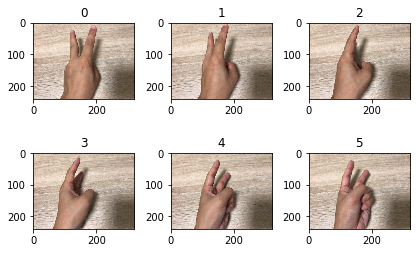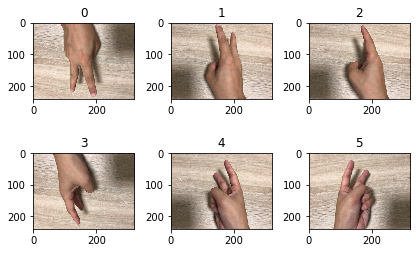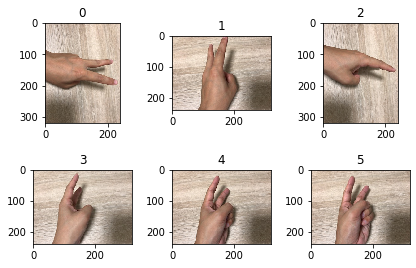# Data Augmentation with PyTorch

Here's how to inflate your data with PyTorch. Regarding PyTorch itself, I wrote an introductory article on my blog before, so please refer to the following if you like.

Introduction to the attention-grabbing deep learning framework "PyTorch"

Please refer to the following article for the reasons for implementing data padding and specific examples.

Image data padding (Data Augmentation) method for improving deep learning accuracy to understand while playing with free materials

If you use Google Colaboratory, you don't need to build an environment and you can do Python machine learning for free.

pytorch_data_preprocessing.ipynb

Click the "Open in Colab" icon in the middle to open it in Google Colab and run it as is.

# Data handling in PyTorch

First of all, let's check the handling of data in PyTorch.

First download the teacher data. The explanation is omitted.

``````!git clone https://github.com/karaage0703/janken_dataset datasets
!rm -rf /content/datasets/.git
``````

The directory has the following structure. Each directory of choki, gu, pa contains pictures of choki, gu, and par hand shapes.

``````datasets
├── choki
├── gu
└── pa
``````

Define `dataset_root_dir` as follows:

``````dataset_root_dir = '/content/datasets'
``````

## Creating a dataset

First, import the required libraries.

``````import torch
from torchvision import transforms, datasets
import matplotlib.pyplot as plt
import PIL
``````

Use ImageFolder to load the images in the folder as a dataset.

``````dataset = datasets.ImageFolder(root=dataset_root_dir)
``````

## Checking the dataset

You can check the contents of dataset with getitem. (# Below is the execution result).

``````print(dataset.__getitem__(0))
print(dataset.__getitem__(100))
print(dataset.__getitem__(150))
# (<PIL.Image.Image image mode=RGB size=320x240 at 0x7F11DB6DC160>, 0)
# (<PIL.Image.Image image mode=RGB size=320x240 at 0x7F11DB6DCF28>, 1)
# (<PIL.Image.Image image mode=RGB size=320x240 at 0x7F12297D2C50>, 2)
``````

To check the contents with matplotlib, follow the steps below.

``````image_numb = 6 #Please specify a multiple of 3
for i in range(0, image_numb):
ax = plt.subplot(image_numb / 3, 3, i + 1)
plt.tight_layout()
ax.set_title(str(i))
plt.imshow(dataset[i])
``````torchvision.transforms In PyTorch, transforms can be used to preprocess various image processing including Data Augmentation.

For typical horizontal / vertical inversion, transforms are written in the following form.

``````data_transform = transforms.Compose([
transforms.RandomHorizontalFlip(),
transforms.RandomVerticalFlip(),
])
``````

After that, if you specify it in the argument of transform of ImageFolder, the dataset with image processing specified by transforms will be defined.

``````dataset_augmentated = datasets.ImageFolder(root=dataset_root_dir, transform=data_transform)
``````

Let's check the data.

``````image_numb = 6 #Please specify a multiple of 3
for i in range(0, image_numb):
ax = plt.subplot(image_numb / 3, 3, i + 1)
plt.tight_layout()
ax.set_title(str(i))
plt.imshow(dataset_augmentated[i])
``````It is upside down.

See the Google Colab notebook for examples of other transforms functions. Techniques such as Random Erasing are also implemented as standard. If you want to know everything, please refer to the official documentation.

# albumentations implementation

This is an easy way to use a library for Data Augmentation called albumentations with PyTorch.

First, install albumations with the following command.

``````! pip install albumentations
``````

Import the required libraries.

``````import albumentations as albu
import numpy as np
from PIL import Image
``````

As with transform, I would like to use Image Folder to inflate data with albumation, but a little technique is required.

You can easily use the functions of albumations with Image Folder by applying the following.

``````albu_transforms = albu.Compose([
albu.RandomRotate90(p=0.5),
albu.RandomGamma(gamma_limit=(85, 115), p=0.2),
])

def albumentations_transform(image, transform=albu_transforms):
if transform:
image_np = np.array(image)
augmented = transform(image=image_np)
image = Image.fromarray(augmented['image'])
return image

data_transform = transforms.Compose([
transforms.Lambda(albumentations_transform),
])

dataset_augmentated = datasets.ImageFolder(root=dataset_root_dir, transform=data_transform)
``````

Let's check the contents of the data.

``````image_numb = 6 #Please specify a multiple of 3
for i in range(0, image_numb):
ax = plt.subplot(image_numb / 3, 3, i + 1)
plt.tight_layout()
ax.set_title(str(i))
plt.imshow(dataset_augmentated[i])
``````You can see that the image processing of albumentations is done.

After a little research, when using albumations, it seems that datasets are often implemented independently without using ImageFolder, but this is a convenient technique when you want to try it easily with ImageFolder.

You can find out what features albumentations have in the Jupyter Notebook on albumentations-examples published on GitHub by @Kazuhito. Become.

Also, @ Kazuhito's Jupyter Notebook is modified to work with Google Colab below, so if you want to actually move it with your own hands, please refer to it.

albumentations_examples.ipynb (Google Colab compatible version)

mixup The following GitHub repository was helpful when using the popular data inflating method mixup with PyTorch because of its performance.

hongyi-zhang/mixup

See the Google Colab notebook for details on how to mix up and how to check the data after mixing up.

pytorch_data_preprocessing.ipynb

In the case of Keras, the following articles may be helpful.

Mixup augmentation in Keras

# Summary

We have summarized how to inflate data (Data Augmentation) with PyTorch and how to check the data. Please let us know if there are more convenient functions or smarter methods.

# Related article

Move and check what you are doing with Data Augmentation of TensorFlow's Object Detection API